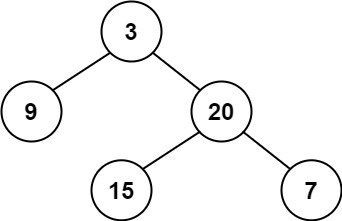| English | 简体中文 |

# 110. Balanced Binary Tree

## Description

Given a binary tree, determine if it is height-balanced.

For this problem, a height-balanced binary tree is defined as:

a binary tree in which the left and right subtrees of every node differ in height by no more than 1.

Example 1:Input: root = [3,9,20,null,null,15,7]
Output: true


Example 2:Input: root = [1,2,2,3,3,null,null,4,4]
Output: false


Example 3:

Input: root = []
Output: true


Constraints:

• The number of nodes in the tree is in the range [0, 5000].
• -104 <= Node.val <= 104